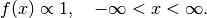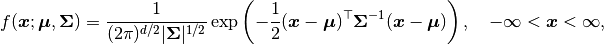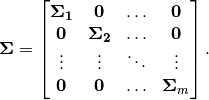# Distributions¶

Given in this section are distributions, as provided by the Distributions  and Mamba packages, supported for the specification of Stochastic nodes. Truncated versions of continuous univariate distributions are also supported.

## Univariate Distributions¶

### Distributions Package Univariate Types¶

The following univariate types from the Distributions package are supported.

Arcsine       DiscreteUniform   InverseGamma       NoncentralChisq            SymTriangularDist
Bernoulli     Edgeworth         InverseGaussian    NoncentralF                TDist
Beta          Epanechnikov      Kolmogorov         NoncentralHypergeometric   TriangularDist
BetaPrime     Erlang            KSDist             NoncentralT                Triweight
Binomial      Exponential       KSOneSided         Normal                     Uniform
Biweight      FDist             Laplace            NormalCanon                VonMises
Categorical   Frechet           Levy               Pareto                     Weibull
Cauchy        Gamma             Logistic           PoissonBinomial
Chi           Geometric         LogNormal          Poisson
Chisq         Gumbel            NegativeBinomial   Rayleigh
Cosine        Hypergeometric    NoncentralBeta     Skellam


### Flat Distribution¶

A Flat distribution is supplied with the degenerate probability density function:Flat()    # Flat distribution


### User-Defined Univariate Distributions¶

New known, unknown, or unnormalized univariate distributions can be created and added to Mamba as subtypes of the Distributions package ContinuousUnivariateDistribution or DiscreteUnivariateDistribution types. Mamba requires only a partial implementation of the method functions described in the full instructions for creating univariate distributions. The specific workflow is given below.

1. Create a quote block for the new distribution. Assign the block a variable name, say extensions, preceded by the @everywhere macro to ensure compatibility when julia is run in multi-processor mode.

2. The Distributions package contains types and method definitions for new distributions. Load the package and import any of its methods (indicated below) that are extended.

3. Declare the new distribution subtype, say D, within the block. Any constructors explicitly defined for the subtype should accept un-typed or abstract-type (Real, AbstractArray, or DenseArray) arguments. Implementing constructors in this way ensures that they will be callable with the Mamba Stochastic and Logical types.

4. Extend/define the following Distributions package methods for the new distribution D.

minimum(d::D)

Return the lower bound of the support of d.

maximum(d::D)

Return the upper bound of the support of d.

logpdf(d::D, x::Real)

Return the normalized or unnomalized log-density evaluated at x.

5. Test the subtype.

6. Add the quote block (new distribution) to Mamba with the following calls.

using Mamba
@everywhere eval(extensions)


Below is a univariate example based on the linear regression model in the Tutorial.

using Distributed

## Define a new univariate Distribution type for Mamba.
## The definition must be placed within an unevaluated quote block.
@everywhere extensions = quote

## Load needed packages and import methods to be extended
using Distributions
import Distributions: minimum, maximum, logpdf

## Type declaration
mutable struct NewUnivarDist <: ContinuousUnivariateDistribution
mu::Float64
sigma::Float64
end

## The following method functions must be implemented

## Minimum and maximum support values
minimum(d::NewUnivarDist) = -Inf
maximum(d::NewUnivarDist) = Inf

## Normalized or unnormalized log-density value
function logpdf(d::NewUnivarDist, x::Real)
-log(d.sigma) - 0.5 * ((x - d.mu) / d.sigma)^2
end

end

## Test the extensions in a temporary module (optional)
module Testing end
Core.eval(Testing, extensions)
d = Testing.NewUnivarDist(0.0, 1.0)
Testing.minimum(d)
Testing.maximum(d)
Testing.insupport(d, 2.0)
Testing.logpdf(d, 2.0)

@everywhere using Mamba
@everywhere eval(extensions)

## Implement a Mamba model using the new distribution
model = Model(

y = Stochastic(1,
(mu, s2) ->
begin
sigma = sqrt(s2)
UnivariateDistribution[
NewUnivarDist(mu[i], sigma) for i in 1:length(mu)
]
end,
false
),

mu = Logical(1,
(xmat, beta) ->
xmat * beta,
false
),

beta = Stochastic(1,
() -> MvNormal(2, sqrt(1000))
),

s2 = Stochastic(
() -> InverseGamma(0.001, 0.001)
)

)

## Sampling Scheme
scheme = [NUTS(:beta),
Slice(:s2, 3.0)]

## Sampling Scheme Assignment
setsamplers!(model, scheme)

## Data
line = Dict{Symbol, Any}(
:x => [1, 2, 3, 4, 5],
:y => [1, 3, 3, 3, 5]
)
line[:xmat] = [ones(5) line[:x]]

## Initial Values
inits = [
Dict{Symbol, Any}(
:y => line[:y],
:beta => rand(Normal(0, 1), 2),
:s2 => rand(Gamma(1, 1))
)
for i in 1:3
]

## MCMC Simulation
sim = mcmc(model, line, inits, 10000, burnin=250, thin=2, chains=3)
describe(sim)


## Multivariate Distributions¶

### Distributions Package Multivariate Types¶

The following multivariate types from the Distributions package are supported.

Dirichlet   MvNormal   MvTDist   Multinomial   MvNormalCanon   VonMisesFisher


### Block-Diagonal Multivariate Normal Distribution¶

A Block-Diagonal Multivariate Normal distribution is supplied with the probability density function:whereBDiagNormal(mu, C)    # multivariate normal with mean vector mu and block-
# diagonal covariance matrix Sigma such that
# length(mu) = dim(Sigma), and Sigma_1 = ... = Sigma_m = C
# for a matrix C or Sigma_1 = C, ..., Sigma_m = C[m]
# for a vector of matrices C.


### User-Defined Multivariate Distributions¶

New known, unknown, or unnormalized multivariate distributions can be created and added to Mamba as subtypes of the Distributions package ContinuousMultivariateDistribution or DiscreteMultivariateDistribution types. Mamba requires only a partial implementation of the method functions described in the full instructions for creating multivariate distributions. The specific workflow is given below.

1. Create a quote block for the new distribution. Assign the block a variable name, say extensions, preceded by the @everywhere macro to ensure compatibility when julia is run in multi-processor mode.

2. The Distributions package contains types and method definitions for new distributions. Load the package and import any of its methods (indicated below) that are extended.

3. Declare the new distribution subtype, say D, within the block. Any constructors explicitly defined for the subtype should accept un-typed or abstract-type (Real, AbstractArray, or DenseArray) arguments. Implementing constructors in this way ensures that they will be callable with the Mamba Stochastic and Logical types.

4. Extend/define the following Distributions package methods for the new distribution D.

length(d::D)

Return the sample space size (dimension) of d.

insupport{T<:Real}(d::D, x::AbstractVector{T})

Return a logical indicating whether x is in the support of d.

_logpdf{T<:Real}(d::D, x::AbstractVector{T})

Return the normalized or unnomalized log-density evaluated at x.

5. Test the subtype.

6. Add the quote block (new distribution) to Mamba with the following calls.

using Mamba
@everywhere eval(extensions)


Below is a multivariate example based on the linear regression model in the Tutorial.

using Distributed

## Define a new multivariate Distribution type for Mamba.
## The definition must be placed within an unevaluated quote block.
@everywhere extensions = quote

## Load needed packages and import methods to be extended
using Distributions
import Distributions: length, insupport, _logpdf

## Type declaration
mutable struct NewMultivarDist <: ContinuousMultivariateDistribution
mu::Vector{Float64}
sigma::Float64
end

## The following method functions must be implemented

## Dimension of the distribution
length(d::NewMultivarDist) = length(d.mu)

## Logical indicating whether x is in the support
function insupport(d::NewMultivarDist, x::AbstractVector{T}) where {T<:Real}
length(d) == length(x) && all(isfinite.(x))
end

## Normalized or unnormalized log-density value
function _logpdf(d::NewMultivarDist, x::AbstractVector{T}) where {T<:Real}
-length(x) * log(d.sigma) - 0.5 * sum(abs2, x - d.mu) / d.sigma^2
end

end

## Test the extensions in a temporary module (optional)
module Testing end
Core.eval(Testing, extensions)
d = Testing.NewMultivarDist([0.0, 0.0], 1.0)
Testing.insupport(d, [2.0, 3.0])
Testing.logpdf(d, [2.0, 3.0])

@everywhere using Mamba
@everywhere eval(extensions)

## Implement a Mamba model using the new distribution
model = Model(

y = Stochastic(1,
(mu, s2) -> NewMultivarDist(mu, sqrt(s2)),
false
),

mu = Logical(1,
(xmat, beta) -> xmat * beta,
false
),

beta = Stochastic(1,
() -> MvNormal(2, sqrt(1000))
),

s2 = Stochastic(
() -> InverseGamma(0.001, 0.001)
)

)

## Sampling Scheme
scheme = [NUTS(:beta),
Slice(:s2, 3.0)]

## Sampling Scheme Assignment
setsamplers!(model, scheme)

## Data
line = Dict{Symbol, Any}(
:x => [1, 2, 3, 4, 5],
:y => [1, 3, 3, 3, 5]
)
line[:xmat] = [ones(5) line[:x]]

## Initial Values
inits = [
Dict{Symbol, Any}(
:y => line[:y],
:beta => rand(Normal(0, 1), 2),
:s2 => rand(Gamma(1, 1))
)
for i in 1:3
]

## MCMC Simulation
sim = mcmc(model, line, inits, 10000, burnin=250, thin=2, chains=3)
describe(sim)


## Matrix-Variate Distributions¶

### Distributions Package Matrix-Variate Types¶

The following matrix-variate types from the Distributions package are supported.

InverseWishart   Wishart